1636334387

# Graph Neural Networks (GNN) for Information Extraction with PyTorch

In this talk, I will provide a primer on a class of deep learning models known as graph neural networks (GNNs).  These models generalize the well-known convolutional neural networks (CNNs) ubiquitous in computer vision, and the approach of the talk will emphasize that analogy.  Specifically, after a quick general recap on graphs, we will see how to think of an image as a graph where nodes represent pixels and edges represent the relationship of being a neighbor.  After expressing the formulas for a CNN in terms of this graph, it is not hard to imagine ways to pass from the graph of an image to arbitrary graphs.  This way, one naturally arrives at a basic GNN architecture.  Other recent advances, for instance graph attention networks, can also be surveyed, time permitting.

We will then survey the existing Python implementations and supporting libraries, with a focus on the PyTorch framework and on the PyTorch Geometric library in particular.

Next, I will turn to applications in the field of NLP, and in particular information extraction.  I will focus on the problem of understanding documents in tabular format.  Unlike regular paragraphs of text, these documents contain more information than just an ordered sequence of words.  We will see how graphs can be used to encode the spatial disposition of words, capturing enough information to allow training deep learning models with good accuracy and generalization capabilities.

#deeplearning #pytorch #gnn #graphneuralnetworks

## Buddha Community1622694240

## Hands-On Guide to PyTorch Geometric (With Python Code)

Released under MIT license, built on PyTorch , PyTorch Geometric(PyG) is a python framework for deep learning on irregular structures like graphs, point clouds and manifolds, a.k.a Geometric Deep Learning  and contains much relational learning and 3D data processing methods. Graph Neural Network (GNN) is one of the widely used representations learning methods but the implementation of it is quite challenging as the throughput of GPU needs to be achieved on highly sparse and irregular data of varying sizes. PyG overcomes this bottleneck by providing dedicated CUDA kernels for sparse data and mini-batch handlers for varying sizes. Methods implemented in PyG framework are supported by both CPU and GPU.

** ﻿Register for AWS ML Fridays** and learn how to make a career in data science.

PyTorch Geometric was submitted as a workshop paper at ICLR 2019, as FAST GRAPH REPRESENTATION LEARNING WITH PYTORCH GEOMETRIC. The framework was developed by Matthias Fey,_ eJan Eric Lenssn_ from TU Dortmund University.

#developers corner #fast graph representation #gcn #geometric deep learning in ai #gnn #graph convulationsl networks #graph neural networks #python #pytorch1636334387

## Graph Neural Networks (GNN) for Information Extraction with PyTorch

In this talk, I will provide a primer on a class of deep learning models known as graph neural networks (GNNs).  These models generalize the well-known convolutional neural networks (CNNs) ubiquitous in computer vision, and the approach of the talk will emphasize that analogy.  Specifically, after a quick general recap on graphs, we will see how to think of an image as a graph where nodes represent pixels and edges represent the relationship of being a neighbor.  After expressing the formulas for a CNN in terms of this graph, it is not hard to imagine ways to pass from the graph of an image to arbitrary graphs.  This way, one naturally arrives at a basic GNN architecture.  Other recent advances, for instance graph attention networks, can also be surveyed, time permitting.

We will then survey the existing Python implementations and supporting libraries, with a focus on the PyTorch framework and on the PyTorch Geometric library in particular.

Next, I will turn to applications in the field of NLP, and in particular information extraction.  I will focus on the problem of understanding documents in tabular format.  Unlike regular paragraphs of text, these documents contain more information than just an ordered sequence of words.  We will see how graphs can be used to encode the spatial disposition of words, capturing enough information to allow training deep learning models with good accuracy and generalization capabilities.

#deeplearning #pytorch #gnn #graphneuralnetworks1595691960

## How Graph Convolutional Networks (GCN) Work

In this post, we’re gonna take a close look at one of the well-known Graph neural networks named GCN. First, we’ll get the intuition to see how it works, then we’ll go deeper into the maths behind it.

## Why Graphs?

Many problems are graphs in true nature. In our world, we see many data are graphs, such as molecules, social networks, and paper citations networks.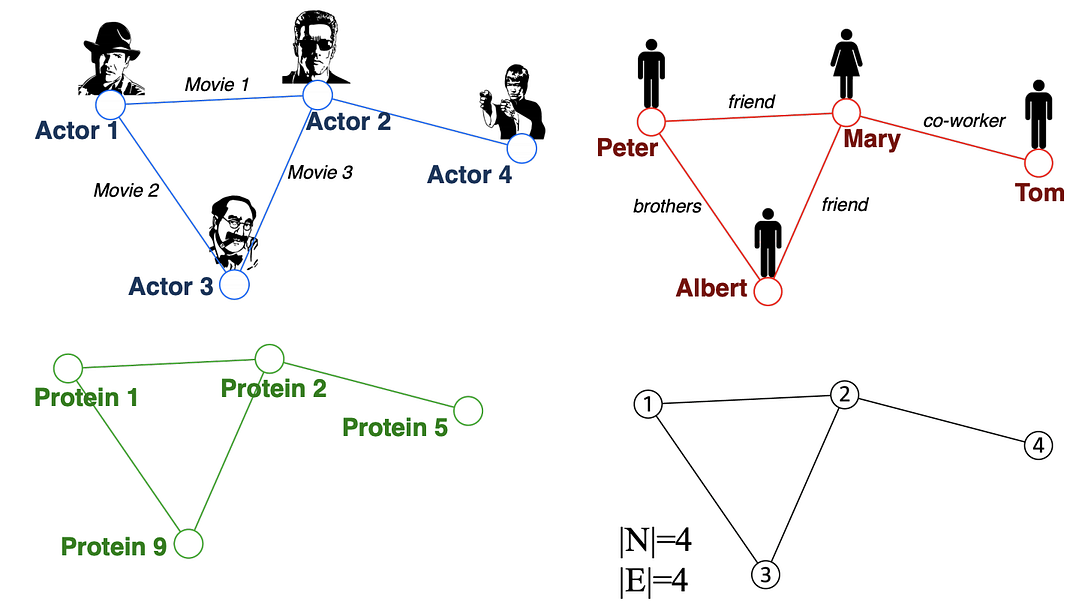Examples of graphs. (Picture from )

• Node classification: Predict a type of a given node
• Community detection: Identify densely linked clusters of nodes
• Network similarity: How similar are two (sub)networks

## Machine Learning Lifecycle

In the graph, we have node features (the data of nodes) and the structure of the graph (how nodes are connected).

For the former, we can easily get the data from each node. But when it comes to the structure, it is not trivial to extract useful information from it. For example, if 2 nodes are close to one another, should we treat them differently to other pairs? How about high and low degree nodes? In fact, each specific task can consume a lot of time and effort just for Feature Engineering, i.e., to distill the structure into our features.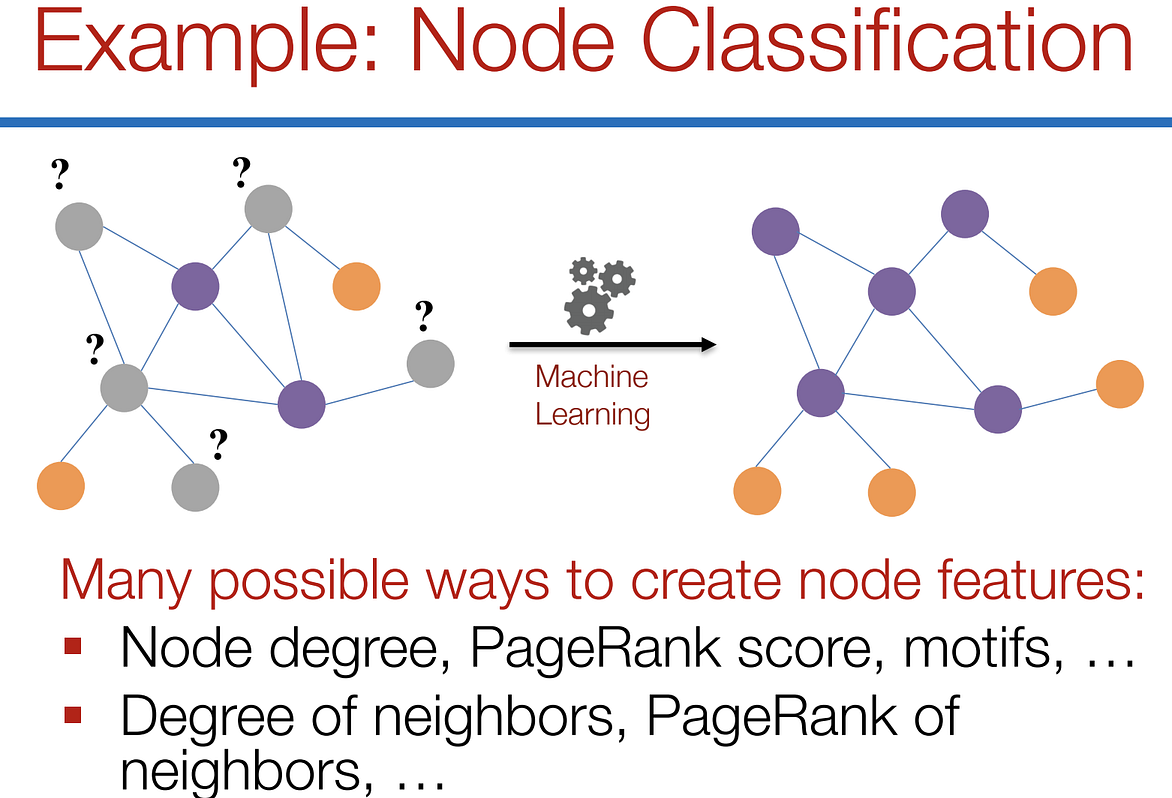Feature engineering on graphs. (Picture from )

It would be much better to somehow get both the node features and the structure as the input, and let the machine to figure out what information is useful by itself.

That’s why we need Graph Representation Learning.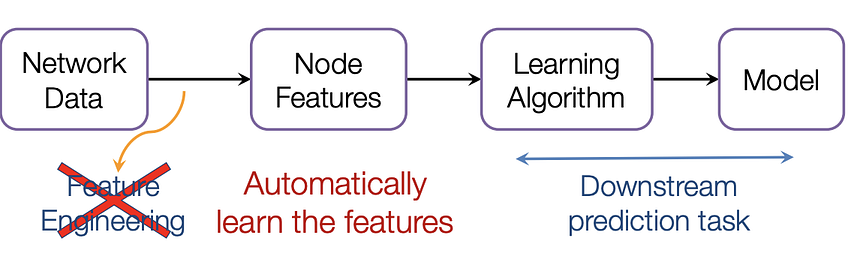We want the graph can learn the “feature engineering” by itself. (Picture from )

# Graph Convolutional Networks (GCNs)

Paper: Semi-supervised Classification with Graph Convolutional Networks(2017) 

GCN is a type of convolutional neural network that can work directly on graphs and take advantage of their structural information.

it solves the problem of classifying nodes (such as documents) in a graph (such as a citation network), where labels are only available for a small subset of nodes (semi-supervised learning).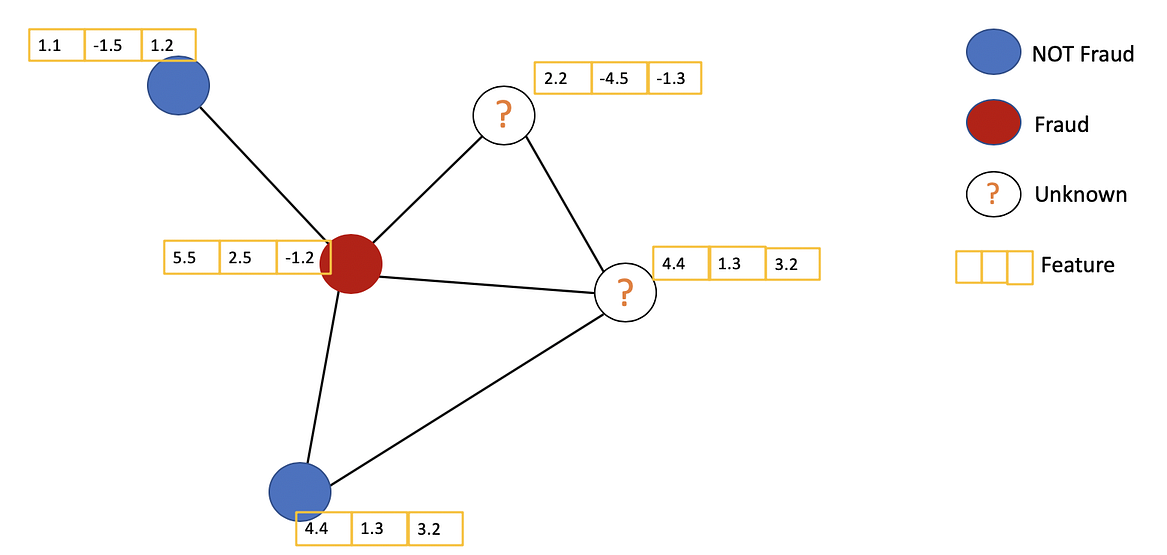Example of Semi-supervised learning on Graphs. Some nodes dont have labels (unknown nodes).

#graph-neural-networks #graph-convolution-network #deep-learning #neural-networks1597632480

## Build your own Neural Network for CIFAR-10 using PyTorch

In 6 simple steps

Neural network seems like a black box to many of us. What happens inside it, how does it happen, how to build your own neural network to classify the images in datasets like MNIST, CIFAR-10 etc. are the questions that keep popping up. Let’s try to understand a Neural Network in brief and jump towards building it for CIFAR-10 dataset. By the end of this article you will have answers to :

1. What are neural networks?
2. How to build a neural network model for cifar-10 dataset by using PyTorch?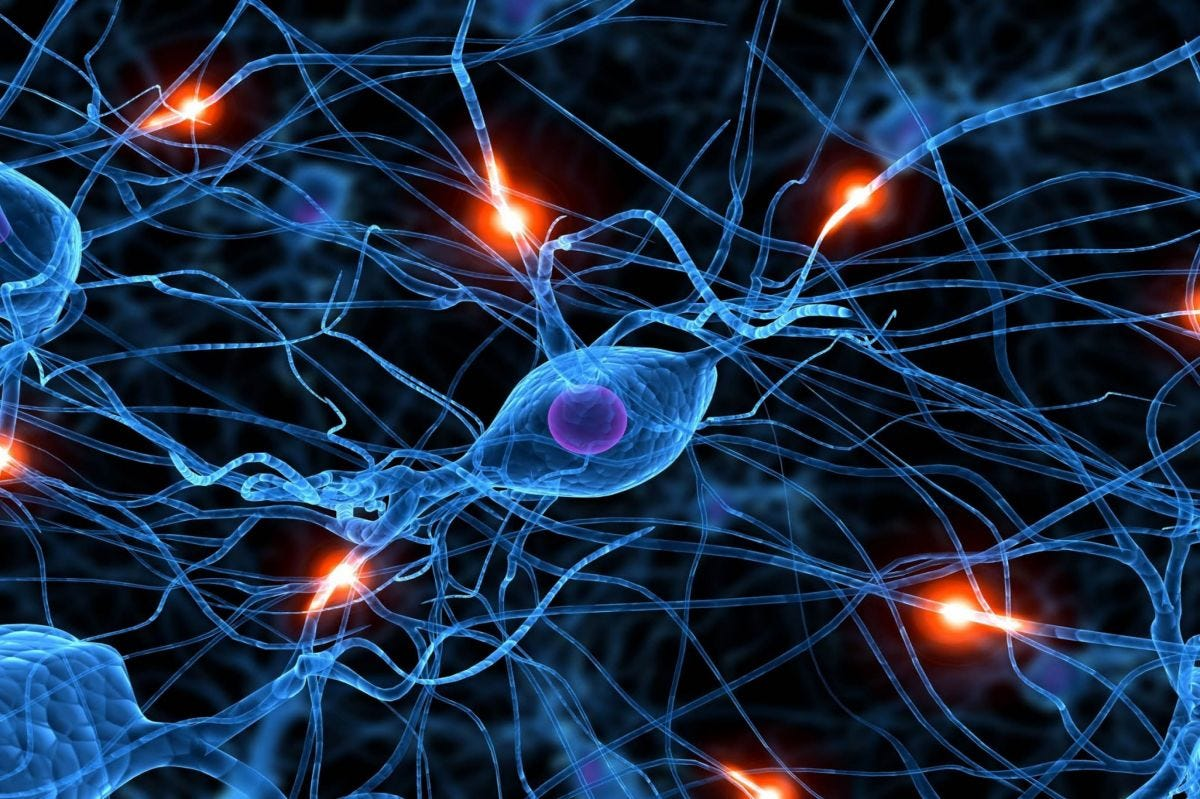### What are neural networks?

Neural networks(NN) are inspired by the human brain. A neuron in a human brain, individually is at rest until it collects signals from others through a structure called dendrites, when the excitation that it receives is sufficiently high, the neuron is fired up(gets activated) and it passes on the information. Artificial neural networks(ANN) are made up of interconnected model/artificial neurons(known as perceptron) that take many weighted inputs , add them up and pass it through a non-linearity to produce an output. Sounds simple!

#neural-networks #machine-learning #pytorch #cifar-10 #neural networks1602838800

## SimGNN: Similarity Computation via Graph Neural Networks

This post will summarize the paper SimGNN which aims for fast graph similarity computation. Graphs are structures that are used to link different entities that we call nodes using relationships called edges. Graphs exist everywhere from bonds between the atoms to friends on Facebook, all these scenarios can be represented as a graph. One of the fundamental graph problems includes finding similarity between graphs. The similarity between graphs can be defined using these metrics :

1. Graph Edit Distance
2. Maximum Common Subgraph

However, currently available algorithms that are used to calculate these metrics have high complexities and it is not yet possible to compute exact GED using these for graphs having more than 16 nodes.

Some ways to compute these metrics are :

1. Pruning verification Framework
2. Approximating the GED in fast and heuristic ways

SimGNN follows another approach to tackle this problem i.e turning similarity computation problem into a learning problem.

Before getting into how SimGNN works, we must know the requirements to be satisfied by this model. It includes :

1. Representation Invariant: Different representations of the same graph should give the same results.
2. **Inductive: **Should be able to predict results for unseen graphs.
3. Learnable: Must work on different similarity metrics like GED and MCS

**SimGNN Approach: **To achieve the above-stated requirements, SimGNN uses two strategies

1. Design Learnable Embedding Function: This maps the graph into an embedding vector, which provides a global summary of a graph. Here, some nodes of importance are selected and used for embedding computation. (less time complexity)
2. Pair-wise node comparison: The above embedding are too coarse, thus further compute the pairwise similarity scores between nodes from the two graphs, from which the histogram features are extracted and combined with the graph level information. (this is a time-consuming strategy)

#graph-edit-distance #machine-learning #graph-neural-networks #graph-convolution-network